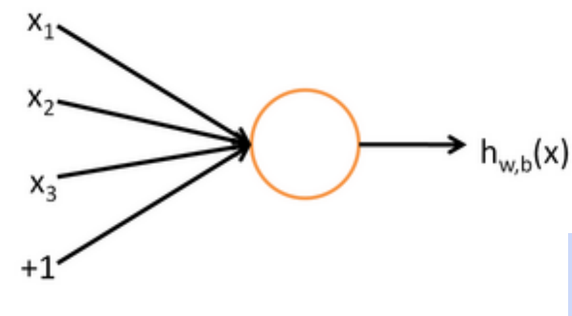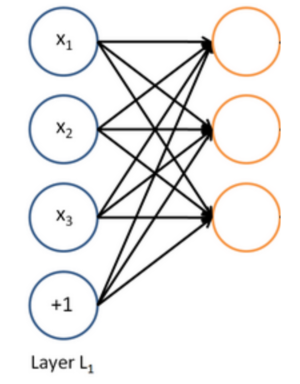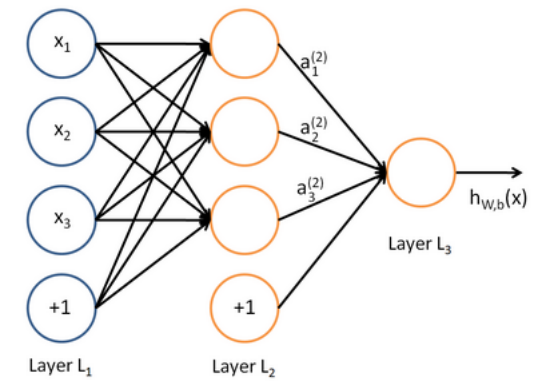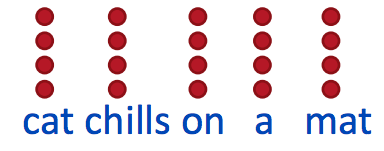# Applying Neural Networks to Natural Language Processing Tasks### Bringing Deep Learning into the field of NLP

Recently, there’s been a lot of advancement in using neural networks and other deep learning algorithms to obtain high performance on a variety of NLP tasks. Traditionally, the bag of words model along with classifiers that use this model, such as the Maximum Entropy Classifier, have been successfully leveraged to make very accurate predictions in NLP tasks such as sentiment analysis. However, with the advent of deep learning research and its applications to NLP, discoveries have been made that improve the accuracy of these methods in primarily two ways: a neural network with several layers of logistic functions, and unsupervised learning to optimize feature selection as a pre-training step.

### How can Neural Networks and other Deep Learning algorithms help?

At its core, deep learning (and neural networks) are all about giving the computer some data, and letting it figure out how it can use this data to come up with features and models to accurately represent complex tasks - such as analyzing a movie review for its sentiment. With more common machine learning algorithms, human-designed features are generally used to model the problem and prediction becomes a task of optimizing weights to minimize a cost function. However, hand crafting features is time consuming, and these human made features tend to either over-represent the general problem and become to specific or are incomplete over the entire problem space.

### Supervised Learning: From Regression to a Neural Network

The Max Entropy classifier, commonly abbreviated to a Maxent classifier, is a common probabilistic model used in NLP. Given some contextual information in a document (in the form of multisets, unigrams, bigrams, etc), this classifier attempts to predict the class label (positive, negative, neutral) for it. This classifier is also used in neural networks, and it’s known as the softmax layer - the final layer (and sometimes only) in the network used for classification. So, we can model a single neuron in a neural network as computing the same function as a max entropy classifier:Here, x is our vector of inputs, the neuron computes the maximum entropy function with parameters w and b and outputs a single result in h.

Then, a neural network with multiple neurons can simply be thought of feeding input to several different classification functions at the same time. A given vector of inputs (x in our above picture) is run through many (as opposed to a single) functions, where each neuron represents a different regression function. As a result, we obtain a vector of outputs:…And you can feed this vector of outputs to another layer of logistic regression functions (or a single function), until you obtain your output, which is the probability that your vector belongs to a certain class:### Applying Neural Networks to Unsupervised Problems in NLP

In NLP, words and their surrounding contexts are pretty important: a word surrounded by relevant context is valuable, while a word surrounded by seemingly irrelevant context is not very valuable. Each word is mapped to a vector defined by its features (which in turn relate to the word’s surrounding context), and neural networks can be used to learn which features maximize a word vector’s score.

A valuable pre-training step for any supervised learning task in NLP (such as classifying restaurant reviews) would be to generate feature vectors that represent words well - as discussed in the beginning of this post, these features are often human-designated. Instead of this, a neural network can be used to learn these features .

The input to such a neural network would be a matrix defined by , for example, a sentence’s word vectors. For example, consider the following phrase and its associated matrix:Our neural network can then be composed of several layers, where each layer sends the previous layer’s output to a function. Training is achieved through back propagation: taking derivates using the chain rule with respect to the weights to optimize these weights. From this, the ideal weights that define our function (which is a composition of many functions) are learned. After training, we now have a method of extracting ideal feature vectors that a given word is mapped to.

This unsupervised neural network is powerful, especially when considered in the context of traditional supervised softmax models. Running this unsupervised network on a large text collection allows input features to be learned rather than human designated, often resulting in better results when these features are fed into a traditional, supervised neural network for classification.

### Recursive Neural Networks

Current researchers are investigating the use of recursive neural networks to learn how sentences are broken down into tree structures. This recursive deep learning network can then successfully learn how to map similar sentences into the same vector space, even though they may be composed of words that mean entirely different things.

If you want to learn about deep learning and neural networks for NLP in detail, I’d highly recommend Stanford’s course on it: Deep Learning for Natural Language Processing.

### Sources

Hidden Layer Neural Networks: Deep Learning, NLP, and Representations

Machine Learning Tutorial: The Max Entropy Text Classifier

Stanford’s Deep Learning Tutorial

CS224d: Deep Learning for Natural Language Processing

Written on August 11, 2016